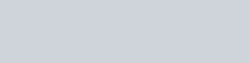Find the length and the equations of the line of shortest distance between the lines given by:
Question:

Find the length and the equations of the line of shortest distance between the lines given by:

$\frac{x-6}{3}=\frac{y-7}{-1}=\frac{z-4}{1}$ and $\frac{x}{-3}=\frac{y+9}{2}=\frac{z-2}{4}$

Solution: